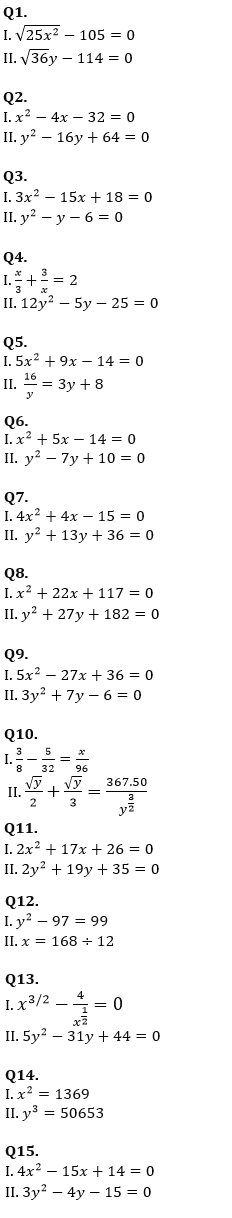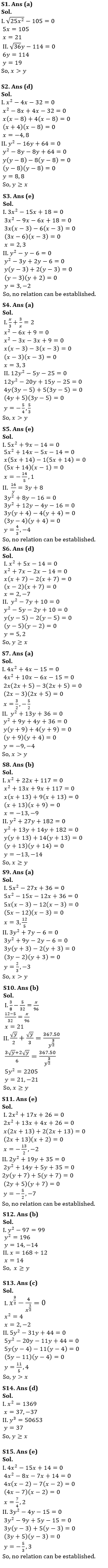Latest Banking jobs   »

# Quantitative Aptitude Quiz For NABARD Grade A 2022- 24th August

Directions (1-15): In each of these questions, two equation (I) and (II) are given. You have to solve both the equations and give answer.                                                                               .
(a) If x > y                                             .                                                                                     .
(b) If x ≥ y
(c) If x < y                  .                                                                                                              .
(d) If x ≤ y
(e) If x = y or no relation can be established between x and y.                                                                .Solutions#### Congratulations!Download Hindu Review of October 2021: Free PDF